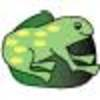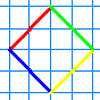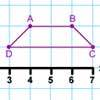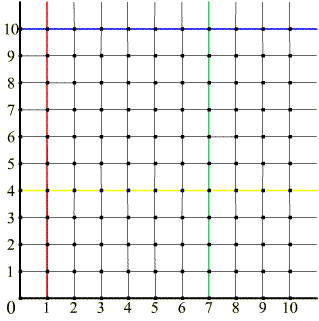#### You may also like### The Lily Pond

Freddie Frog visits as many of the leaves as he can on the way to see Sammy Snail but only visits each lily leaf once. Which is the best way for him to go?### A Cartesian Puzzle

Find the missing coordinates which will form these eight quadrilaterals. These coordinates themselves will then form a shape with rotational and line symmetry.### Transformation Tease

What are the coordinates of this shape after it has been transformed in the ways described? Compare these with the original coordinates. What do you notice about the numbers?

# What Are You Plotting?

##### Age 7 to 11Challenge Level

On a set of axes (like the ones below), plot all the points which have an x coordinate of 3.
What do you notice about the position of all these points?

Now plot all the points which have a y coordinate of 5.
What do you notice about the position of these points?

Give the coordinates at the point where the two lines you have drawn meet.
We call this the point of intersection of the lines.Now look at the set of axes above. What do all the points on the blue line have in common?
Name 3 points on the green line.
What are the coordinates of the point which is on both the red and yellow lines?
Which line would the point (17,4) be on?